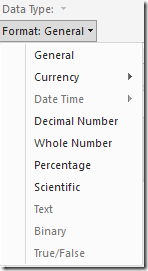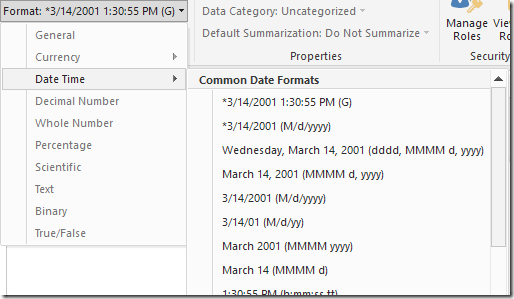If you want to format a measure as date in Power BI, you need an expression returning a date/time data type. This is different from what you can do in Power Pivot and in SSAS Tabular, where you can format any numeric expression using a date and/or time format, and the conversion is implicitly done to perform such a visualization.

The problem might be not very common, because you have a numeric expression only when you start manipulating a date without using the dedicated DAX functions to do that. A date is a floating point number where the integer part is the distance in days since December 30, 1899, and the decimal part is the fraction of a day. In the following examples I will use NOW() to obtain a date and time, but you might have any other expression operating on your data.

This measure in Power BI returns a date/time, and can be formatted using any “Date Time” format:

Measure := NOW ()

You can remove the time by truncating the number, obtaining only the day with the time corresponding to 12:00am:

Measure := TRUNC ( NOW () )

At this point, if you try to change the format of the measure, the “Date Time” format is disabled:In DAX there is no type conversion operator to date/time. However, you can sum the numeric expression that you have to a recognized date/time value. If you add the equivalent of 0 (corresponding to December 30, 1899), your final data type is a date/time. The following expression returns a date/time data type corresponding to the current day.

Measure := TRUNC ( NOW() ) + DATE ( 1899, 12, 30 )

The format of the measure now displays only Date Time formats.Please note that for the specific example used in this post, you can use the DAX function TODAY(), which returns the current date without the time. The function NOW has been used only to represent any date/time expression that you might obtain from other DAX expressions over your data.

Thanks to Jeffrey Wang for providing me the hint used in the technique I described in this blog post.

NOWReturns the current date and time in datetime format.

`NOW ( )`

TRUNCTruncates a number to an integer by removing the decimal, or fractional, part of the number.

`TRUNC ( <Number> [, <NumberOfDigits>] )`

DATEReturns the specified date in datetime format.

`DATE ( <Year>, <Month>, <Day> )`

TODAYReturns the current date in datetime format.

`TODAY ( )`